### D.84 Band gap ex­pla­na­tion de­riva­tions

To see math­e­mat­i­cally how the re­sults of note {N.9} were ob­tained re­quires knowl­edge of lin­ear al­ge­bra. If you are un­aware of it, def­i­nitely skip the be­low de­riva­tion.

First de­fine the “growth ma­trix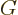that gives the val­ues of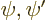at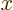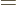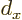given the val­ues at0: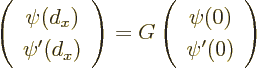Sim­ply take the ini­tial con­di­tions to be 1,0 and 0,1 re­spec­tively, and find the so­lu­tions atto find the two columns of.

Since the po­ten­tial is the same in all atomic cell, ma­trixde­scribes the change over any cell, not just the first one. And for a pe­ri­odic so­lu­tion for a box with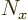atoms, af­terap­pli­ca­tions ofthe orig­i­nal val­ues ofmust be ob­tained. Ac­cord­ing to lin­ear al­ge­bra, and as­sum­ing that the two eigen­val­ues ofare un­equal, that means that at least one eigen­value ofraised to the powermust be 1.

Now ma­trixmust have unit de­ter­mi­nant, be­cause for the two ba­sic so­lu­tions with 1,0 and 0,1 ini­tial con­di­tions,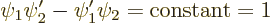for all. The quan­tity in the left hand side is called the Wron­skian of the so­lu­tions. To ver­ify that it is in­deed con­stant, take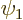times the Hamil­ton­ian eigen­value prob­lem for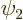mi­nustimes the one forto get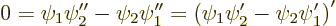Ac­cord­ing to lin­ear al­ge­bra, ifhas unit de­ter­mi­nant then the prod­uct of its two eigen­val­ues is 1. There­fore, if its eigen­val­ues are un­equal and real, their mag­ni­tude is un­equal to 1. One will be less than 1 in mag­ni­tude and the other greater than 1. Nei­ther can pro­duce 1 when raised to the power, so there are no pe­ri­odic so­lu­tions. En­er­gies that pro­duce such ma­tri­cesare in the band gaps.

If the eigen­val­ues ofare com­plex con­ju­gates, they must have mag­ni­tude 1. In that case, the eigen­val­ues can al­ways be writ­ten in the form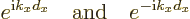for some value of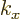. For ei­ther eigen­value raised to the powerto pro­duce 1,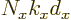must be a whole mul­ti­ple of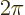. That gives the same wave num­ber val­ues as for the free-elec­tron gas.

To see when the eigen­val­ues ofhave the right form, con­sider the sum of the eigen­val­ues. This sum is called the trace. If the eigen­val­ues are real and un­equal, and their prod­uct is 1, then the trace ofmust be greater than 2 in mag­ni­tude. (One way of see­ing that for pos­i­tive eigen­val­ues is to mul­ti­ply out the ex­pres­sion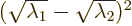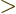0. For neg­a­tive ones, add two mi­nus signs in the square roots.) Con­versely, when the eigen­val­ues are com­plex con­ju­gates, their sum equals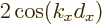ac­cord­ing to the Euler for­mula (2.5). That is less than 2 in mag­ni­tude. So the con­di­tion for valid pe­ri­odic eigen­func­tions be­comes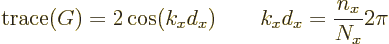From the fact that pe­ri­odic so­lu­tions with twice the crys­tal pe­riod ex­ist, (the ones at the band gaps), it is seen that the val­ues of the trace must be such that the co­sine runs through the en­tire gamut of val­ues. In­deed when the trace is plot­ted as a func­tion of the en­ergy, it os­cil­lates in value be­tween min­ima less than -2 and max­ima greater than 2. Each seg­ment be­tween ad­ja­cent min­ima and max­ima pro­duces one en­ergy band. At the gap en­er­gies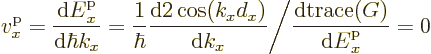be­cause the co­sine is at its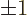ex­trema at the gap en­er­gies. So the ve­loc­ity be­comes zero at the ends of the bands.

Iden­ti­fi­ca­tion of the eigen­func­tions us­ing the growth ma­trixis read­ily put on a com­puter. A canned zero finder can be used to find the en­er­gies cor­re­spond­ing to the al­lowed val­ues of the trace.